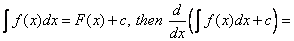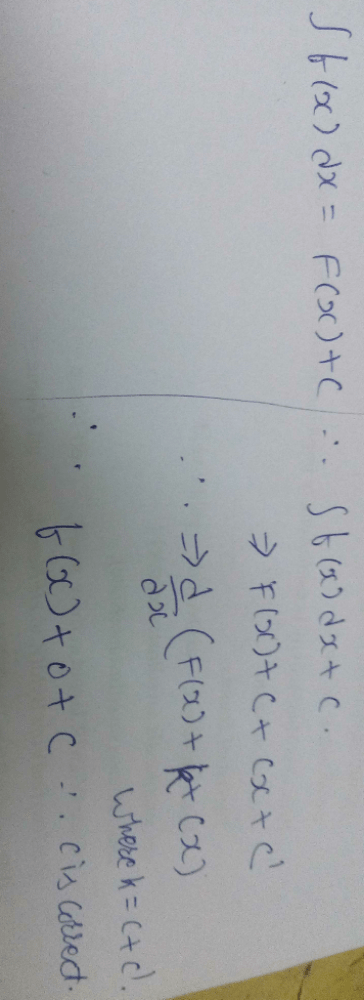Courses

# Can you explain the answer of this question below:A:F(x) + cB:f(x)C:f(x) + cD:None of the aboveThe answer is d.

## JEE Question

By Narendra Mahajan · Aug 12, 2018 ·JEE
Aaryan Kataria answered Aug 12, 2018
See, as you know that integral of f(x) with respect to dx will be F(x) + c where c is a constant. now differentiating it will give F'(x) + 0 as derivative of a constant is 0. Hence the answer to this question is none of the above

Ramachal Singh answered Jun 04, 2018
Non of these

Hitesh Kumar answered Oct 31, 2019
B

Md Saif Ansari answered Jul 05, 2019
First you integrate something and then differentiate it, you will get the same result!

Sunand Raval answered Nov 05, 2019Piyush Raj answered Aug 30, 2019
According to me correct option is C

Mubeen Tambe answered Sep 01, 2019
D by dx of fx plus C is f'x plus 0

Harshvardhan Rana answered Nov 20, 2019
Logic